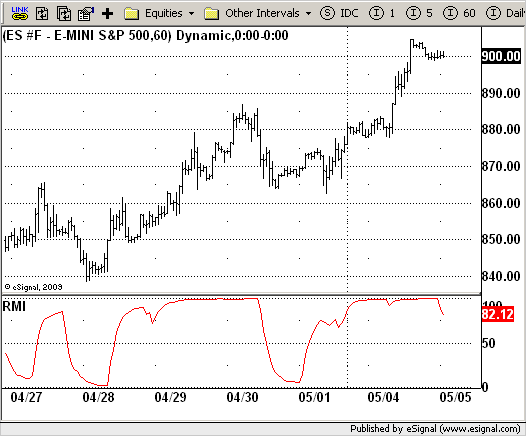# Relative Momentum Index (RMI) - Alternative

ICE Data Services -

RMI_Alternative.efs
EFSLibrary - Discussion Board

File Name: RMI_Alternative.efs

Description:
Relative Momentum Index (RMI) - Alternative

Formula Parameters:

• Length : 5
• Y : 10

Notes:

This is an alternative RMI version.
The Relative Momentum Index (RMI) was developed by Roger Altman. Impressed with the Relative Strength Index's sensitivity to the number of look-back periods, yet frustrated with it's inconsistent oscillation between defined overbought and oversold levels, Mr. Altman added a momentum component to the RSI.
As mentioned, the RMI is a variation of the RSI indicator. Instead of counting up and down days from close to close as the RSI does, the RMI counts up and down days from the close relative to the close x-days ago where x is not necessarily 1 as required by the RSI). So as the name of the indicator reflects, "momentum" is substituted for "strength".

RMI_Alternative.efsEFS Code:

```/*********************************
Provided By:
eSignal (Copyright c eSignal), a division of Interactive Data
Formula Script (EFS) is for educational purposes only and may be
modified and saved under a new file name.  eSignal is not responsible
for the functionality once modified.  eSignal reserves the right
to modify and overwrite this EFS file with each new release.

Description:
Relative Momentum Index (RMI) - Alternative

Version:            1.0  05/04/2009

Formula Parameters:                     Default:
Length                              5
Y                                   10

Notes:
This is an alternative RMI version.
The Relative Momentum Index (RMI) was developed by Roger Altman. Impressed
with the Relative Strength Index's sensitivity to the number of look-back
periods, yet frustrated with it's inconsistent oscillation between defined
overbought and oversold levels, Mr. Altman added a momentum component to the RSI.
As mentioned, the RMI is a variation of the RSI indicator. Instead of counting
up and down days from close to close as the RSI does, the RMI counts up and down
days from the close relative to the close x-days ago where x is not necessarily
1 as required by the RSI). So as the name of the indicator reflects, "momentum"
is substituted for "strength".
**********************************/
var fpArray = new Array();
var bInit = false;

function preMain() {
setStudyTitle("RMI");
setCursorLabelName("RMI", 0);
setDefaultBarFgColor(Color.red, 0);
setStudyMax(101);
setStudyMin(-1);
var x = 0;
fpArray[x] = new FunctionParameter("Length", FunctionParameter.NUMBER);
with(fpArray[x++]) {
setLowerLimit(1);
setDefault(5);
}
fpArray[x] = new FunctionParameter("Y", FunctionParameter.NUMBER);
with(fpArray[x++]) {
setLowerLimit(1);
setDefault(10);
}
}

var xRMI_a = null;

function main(Length, Y) {
var nBarState = getBarState();
var nRMI_a = 0;
if (nBarState == BARSTATE_ALLBARS) {
if (Length == null) Length = 5;
if (Y == null) Y = 10;
}
if (bInit == false) {
xRMI_a = efsInternal("Calc_RMI_a", Length, Y);
bInit = true;
}
nRMI_a = xRMI_a.getValue(0);
if (nRMI_a == null) return;
return nRMI_a;
}

var bSecondInit = false;
var xMOM = null;
var	xUpAvg = null;
var xDownAvg = null;

function Calc_RMI_a(Length, Y) {
var nRes = 0;
var nUpAvg = 0;
var nDownAvg = 0;
if (bSecondInit == false) {
xMOM = mom(Y);
xUpAvg = efsInternal("Calc_UpAvg", Length, xMOM);
xDownAvg = efsInternal("Calc_DownAvg", Length, xMOM);
bSecondInit = true;
}
nUpAvg = xUpAvg.getValue(0);
nDownAvg = xDownAvg.getValue(0);
if (nUpAvg == null || nDownAvg == null) return;
if(nUpAvg + nDownAvg != 0) {
nRes = 100 * nUpAvg / (nUpAvg + nDownAvg);
}
return nRes;
}

function Calc_UpAvg(Length, xMOM) {
var nRes = 0;
var nRef = ref(-1);
var nVal = xMOM.getValue(0);
if (xMOM.getValue(-1) == null) return;
if(nVal < 0) nVal = 0;
nRes = (nRef * (Length - 1) + nVal) / Length;
return nRes;
}

function Calc_DownAvg(Length, xMOM) {
var nRes = 0;
var nRef = ref(-1);
var nVal = xMOM.getValue(0);
if (xMOM.getValue(-1) == null) return;
if(nVal >= 0) {
nVal = 0;
} else {
nVal = -nVal;
}
nRes = (nRef * (Length - 1) + nVal) / Length;
return nRes;
}```